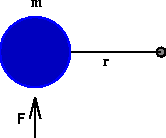# Derivation of the Moment of Inertia FormulaSuppose a particle of mass m is attached to a pivot by a thin rod of length r . As the particle travels around the circle, we know that the distance it travels is equal to the angle the rod sweeps out measured in radians multiplied by the radius r . Differentiating twice shows that

a = r A

where A is the angular acceleration (i.e. the rate at which the angular velocity of the rod is changing) and a is the instantaneous linear acceleration the particle experiences out on the circle.

By Newton's second law for linear motion, if we apply a force F to the particle, then F = m a . On the other hand, since we have a rotating system, we would like to work with torque , instead of force, so we multiply both sides of the equation by r . Then

T = F r = m r a .

Finally, we use the equation derived about, to convert from linear acceleration to angular acceleration:

T = m r a = m r (A r ).

Rearranging terms gives the desired formula T = (m r ^2) A.